Name:    MHF4U - 4.1Quiz

True/False
Indicate whether the statement is true or false.

1.

The radian is an alternative unit to the degree for angle measurement.

Multiple Choice
Identify the choice that best completes the statement or answers the question.

2.

The exact radian measure for an angle of 30° is
 a.c.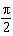b.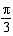d.3.

The exact radian measure for an angle of 15° is
 a.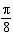c.b.d.4.

The exact radian measure for an angle of 135° is
 a.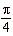c.b.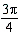d.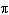5.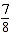of a revolution represents how many radians?
 a.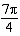c.b. 315 d. 157.5

6.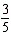of a revolution represents approximately how many radians?
 a. 6.28 c. 3.77 b. 3.14 d. 108

7.

Determine the exact degree measure for.
 a.c.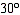b.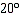d.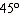8.

Determine the exact degree measure for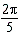.
 a.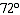c.b.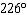d.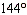9.

Determine the approximate degree measure for an angle of 1.32 radians.
 a. 136.4° c. 75.6° b. 4.2° d. 2.4°

10.

Determine the approximate degree measure for an angle of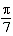radians.
 a. 25.7° c. 0.4° b. 51.4° d. 22.0°